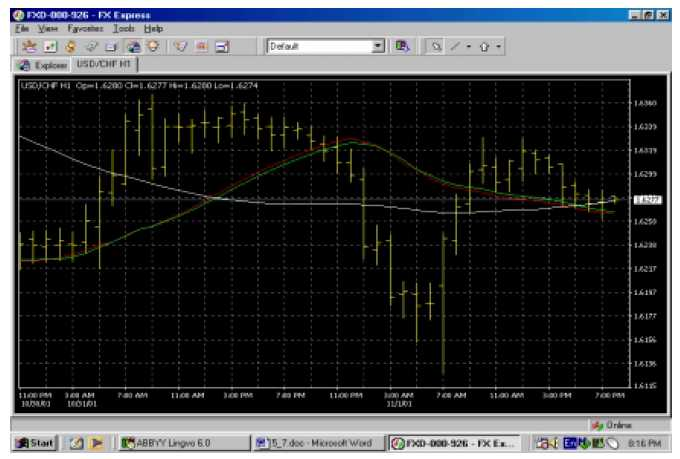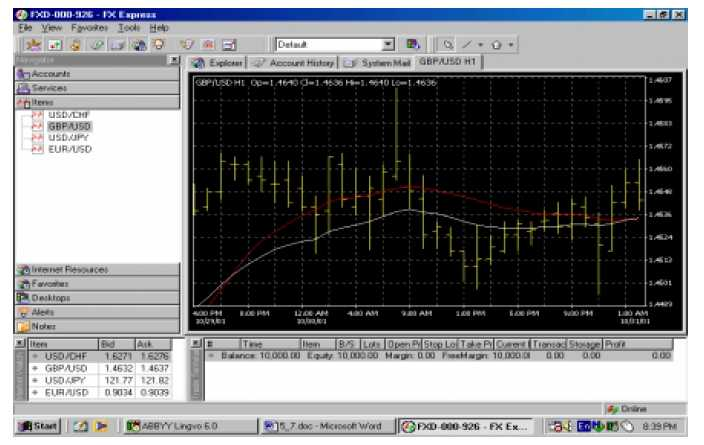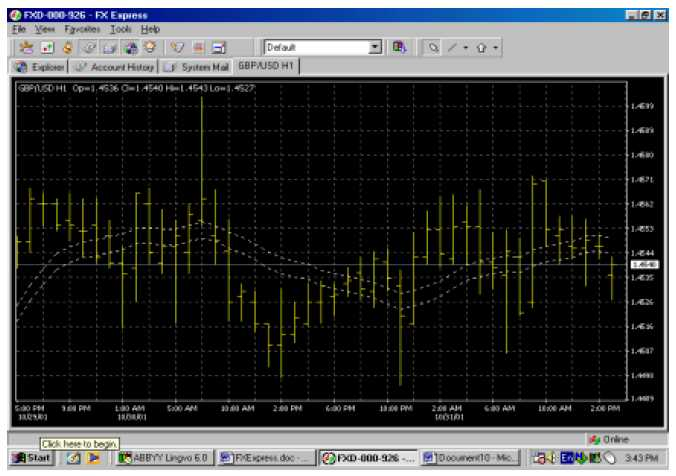# Technical Analysis – Mathematical Trading Methods (Indicators)

No comment 597 views

The mathematical trading methods provide a more objective view of price activity. In addition, these methods tend to provide signals prior to their occurrence on the currency charts. The tools of the mathematical methods are moving averages and oscillators.

## Moving Averages

A moving average is an average of a predetermined number of prices over a number of days, divided by the number of entries. The higher the number of days in the average, the smoother the line is. A moving average makes it easier to visualize currency activity without daily statistical noise. It is a common tool in technical analysis and is used either by itself or as an oscillator.

As one can see from Figure 5.35., a moving average has a smoother line than the underlying currency. The daily closing price is commonly included in the moving averages. The average may also be based on the midrange level or on a daily average of the high, low, and closing prices.Figure 5.35. Examples of three simple moving averages—5-day (white), 20-day (red) and 60-day (green)

It is important to observe that the moving average is a follower rather than a leader. Its signals occur after the new movement has started, not before.

There are three types of moving averages:

1. The simple moving average or arithmetic mean.
2. The linearly weighted moving average.
3. The exponentially smoothed moving average.

As described, the simple moving average or arithmetic mean is the average of a predetermined number of prices over a number of days, divided by the number of entries. Traders have the option of using a linearly weighted moving average (See Figure 5.36.). This type of average assigns more weight to the more recent closings. This is achieved by multiplying the last day’s price by one, and each closer day by an increasing consecutive number. In our previous example, the fourth day’s price is multiplied by 1, the third by 2, the second by 3, and the last one by 4; then the fourth day’s price is deducted. The new sum is divided by 9, which is the sum of its multipliers.Figure 5.36. Example of a 20-day simple moving average (red) as compared to a 20-day weighted moving average (white)

The most sophisticated moving average available is the exponentially smoothed moving average. (See Figure 5.37.) In addition to assigning different weights to the previous prices, the exponentially smoothed moving average also takes into account the previous price information of the underlying currency.Figure 5.37. Example of a 20-day simple moving average (red) as compared to a 20-day exponential moving average (white)

### Trading Signals of Moving Averages

Single moving averages are frequently used as price and time filters. As a price filter, a short-term moving average has to be cleared by the currency closing price, the entire daily range, or a certain percentage (chosen at the discretion of the trader). The envelope model (See Figure 5.38.) serves as a price filter. It consists of a short-term (perhaps 5-day) closing price based moving average to which a small percentage (2 percent is suggested for foreign currencies.) are added and substracted. The two winding parallel lines above and below the moving average will create a band bordering most price fluctuations.

When the upper band is penetrated, a selling signal occurs. When the lower band is penetrated, a buying signal occurs. Because the signals generated by the envelope model are very short-term and they occur many times against the ongoing direction of the market, speed of execution is paramount. The high-low band is set up the same way, except that the moving average is based on the high and low prices. As a time filter, a short number of days may be used to avoid any false signals.Figure 5.38. An envelope model define the edges of the band. A close above the upper band sends a buying signal and one below the lower band gives a selling signal

Usually traders choose a number of averages to use with a currency. A suggested number is three, as more signals may be available. It may be helpful to use intervals that better encompass short-term, medium-term, and long-term periods, to arrive at a more complex set of signals. Some of the more popular periods are 4, 9, and 18 days; 5, 20, and 60 days; and 7, 21, and 90 days. Unless you focus on a specific combination of moving averages (for instance, 4, 9, and 18 days), the exact number of days for each of the averages is less important, as long as they are spaced far enough apart from each other to avoid insignificant signals.

A buying signal on a two-moving average combination occurs when the shorter term of two consecutive averages intersects the longer one upward. A selling signal occurs when the reverse happens, and the longer of two consecutive averages intersects the shorter one downward. (See Figure 5.39.)

## Oscillators

Oscillators are designed to provide signals regarding overbought and oversold conditions. Their signals are mostly useful at the extremes of their scales and are triggered when a divergence occurs between the price of the underlying currency and the oscillator. Crossing the zero line, when applicable, usually generates direction signals. Examples of the major types of oscillators are moving averages convergence-divergence (MACD), momentum and relative strength index (RSI).Figure 5.39. Examples of a sell signal (first and third crossovers) and a buy signals (second crossover) provided by the 5-day (red) and 20-day (white) moving averages

## Stochastics

Stochastics generate trading signals before they appear in the price itself. Its concept is based on observations that, as the market gets high, the closing prices tend to approach the daily highs; whereas in a bottoming market, the closing prices tend to draw near the daily lows.

The oscillator consists of two lines called %K and %D. Visualize %K as the plotted instrument, and %D as its moving average.

The formulas for calculating the stochastics are:

%K = [(CCL -L9)I(H9 – L9)] * 100, where

CCL = current closing price

L9 – the lowest low of the past 9 days

H9 – the highest high of the past 9 days

and

%D=(H3/L3~) * 100,

where            H3 = the three-day sum of (CCL – L9)

L3 = the three-day sum of (H9 – L9)

The resulting lines are plotted on a 1 to 100 scale, with overbought and oversold warning signals at 70 percent and 30 percent, respectively. The buying (bullish reversal) signals occur under 10 percent, and conversely the selling (bearish reversal) signals come into play above 90 percent after the currency turns. (See Figure 5.40.) In addition to these signals, the oscillator-currency price divergence generates significant signals.

The intersection of the %D and %K lines generates further trading signals.

There are two types of intersections between the %D and %K lines:

1. The left crossing, when the %K line crosses prior to the peak of the %D line.
2. The right crossing, when the %K line occurs after the peak of the %D line.

## Moving Average Convergence-Divergence (MACD)

The moving average convergence-divergence (MACD) oscillator, developed by Gerald Appel, is built on exponentially smoothed moving aver ages. The MACD consists of two exponential moving averages that are plotted against the zero line. The zero line represents the times the values of the two moving averages are identical.

In addition to the signals generated by the averages’ intersection with the zero line and by divergence, additional signals occur as the shorter average line intersects the longer average line. The buying signal is displayed by an upward crossover, and the selling signal by a downward crossover. (See Figure 5.41.)

## Momentum

Momentum is an oscillator designed to measure the rate of price change, not the actual price level. This oscillator consists of the net difference between the current closing price and the oldest closing price from a predetermined period.

The formula for calculating the momentum (M) is:

M =  CCP-OCP, where

CCP – current closing price

OCP – old closing price for the predetermined period.

The new values thus obtained will be either positive or negative numbers, and they will be plotted around the zero line. At extreme positive values, momentum suggests an overbought condition, whereas at extreme negative values, the indication is an oversold condition. (See Figure 5.42.) The momentum is measured on an open scale around the zero line.

This may create potential problems when a trader must figure out exactly what an extreme overbought or oversold condition means. On the simplest level, the relativity of the situation may be addressed by analyzing the previous historical data and determining the approximate levels that delineate the extremes. The shorter the number of days included in the calculations, the more responsive the momentum will be to short-term fluctuations, and vice versa. The signals triggered by the crossing of the zero line remain in effect. However, they should be followed only when they are consistent with the ongoing trend.

## The Relative Strength Index (RSI)

The relative strength index is a popular oscillator devised by Welles Wilder. The RSI measures the relative changes between the higher and lower closing prices. (See Figure 5.43.)

The formula for calculating the RSI is:

Л5/=100-[100/(1+RS)], where

RS – (average of X days up closes/average of X days down closes);

X – predetermined number of days The original number of days, as used by its author, was 14 days. Currently, a 9-day period is more popular.

The RSI is plotted on a 0 to 100 scale. The 70 and 30 values are use as warning signals, whereas values above 85 indicate an overbought condition (selling signal) and values under 15 indicate an oversold condition (buying signal.) Wilder identified the RSI’s forte as its divergence versus the underlying price.

## Rate of Change (ROC)

The rate of change is another version of the momentum oscillator. The difference consists in the fact that, while the momentum’s formula is based on subtracting the oldest closing price from the most recent, the ROC’s formula is based on dividing the oldest closing price into the most recent one. (See Figure 5.44.)

ROC = (CCP/OCP) * 100, where

CCP – current closing price;

OCP = old closing price for the predetermined period Larry Williams %R.

## The Larry Williams %R

The Larry Williams %R is a version of the stochastics oscillator. It consists of the difference between the high price of a predetermined number of days and the current closing price, which difference in turn is divided by the total range. This oscillator is plotted on a reversed 0 to 100 scale.

Therefore, the bullish reversal signals occur at under 80 percent, and the bearish signals appear at above 20 percent. The interpretations are similar to those discussed under stochastics. (See Figure 5.45.)

## Commodity Channel Index (CCI)

The commodity channel index was developed by Donald Lambert. It consists of the difference between the mean price of the currency and the average of the mean price over a predetermined period of time (See Figure 5.46.). A buying signal is generated when the price exceeds the upper (+100) line, and a selling signal occurs when the price dips under the lower (-100) line. (See Figure 5.46.)

## Bollinger Bands

The Bollinger bands combine a moving average with the instrument’s volatility. The bands were designed to gauge whether prices are high or low on a relative basis via volatility. The two are plotted two standard deviations above and below a 20-day simple moving average.

The bands look a lot like an expanding and contracting envelope model.

When the band contracts drastically, the signal is that volatility is low and thus likely to expand in the near future. An additional signal is a succession of two top formations, one outside the band followed by one inside. If it occurs above the band, it is a selling signal. When it occurs below the band, it is a buying signal. (See Figure 5.47.)

## The Parabolic System (SAR)

The parabolic system is a stop-loss system based on price and time. The system was devised to supplement the inadvertent gaps of the other trend-following systems. The name of the system is derived from its parabolic shape, which follows the price gyrations. It is represented by a dotted line.

When the parabola is placed under the price, it suggests a long position. Conversely, when placed above the price, the parabola indicates a short position. (See Figure 5.48.) The parabolic system can be used with oscillators. SAR stands for stop and reverse. The stop moves daily in the direction of the new trend. The built-in acceleration factor pushes the SAR to catch up with the currency price. If the new trend fails, the SAR signal will be generated.

## The Directional Movement Index (DMI)

The directional movement index provides a signal of trend presence in the market. The line simply rates the price directional movement on a scale of 0 to 100. The higher the number, the better the trend potential of a movement, and vice versa. (See Figure 5.49.) This system can be used by itself or as a filter to the SAR system.

Traders use different combinations of technical tools in their daily trading and analysis. Some of the more popular oscillators are shown in Figure 5.50.Forex Signal 30 is the best forex system since 2009 and has been used by thousands of traders from around the world to generate profit in forex trading. This system is created by our team of Brilliant Forex Signal Team, this system is made as simple as possible for beginner and professional traders.